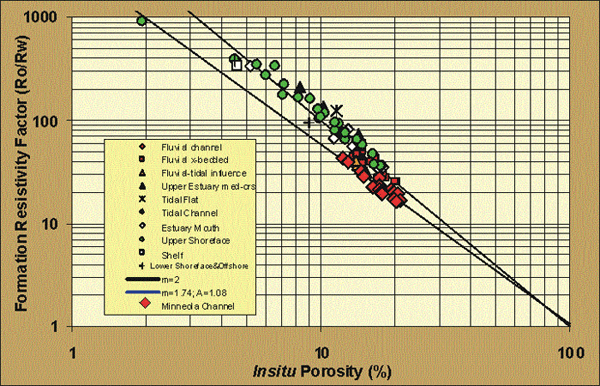Core Petrophysical Properties

Archie Cementation Exponent

Wireline log water saturations were interpreted using the available resistivity logs and the Archie equation for water saturation calculations. Previous analysis of Morrow sandstones has shown that the cementation exponent, m=1.81, and intercept, a=1, for channel sandstones and m=2 and a=1, for other lithofacies (Figure). For the Morrow in the Minneola Unit, average m=1.78+0.09 for a=1 for the channel sands, or alternately, linear regression analysis of the trend indicates that m=1.74 and a=1.08. Other lithofacies exhibit an average m=2.